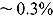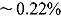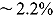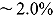The Real-time Analysis for Particle transport and In-situ Detection (RAPID) code uses a unique, extremely fast, fission matrix–based methodology to compute the eigenvalue, and three-dimensional, pinwise fission source distribution for reactor, spent fuel pool, and spent fuel cask problems. In this paper, the RAPID fission matrix method is described and analyzed for application to several large pressurized water reactor problems, based on the Organisation for Economic Co-operation and Development/Nuclear Energy Agency Monte Carlo Performance Benchmark problem. In the RAPID methodology, fission matrix coefficients precalculated using the Serpent Monte Carlo code, are then coupled together and solved for different core arrangements. A boundary correction method is used to obtain more accurate fission matrix values near the radial and axial reflectors. Eigenvalues and fission source distributions are compared between RAPID and Serpent reference calculations. In most cases, the eigenvalue differences between methods are less than 10 pcm. For a uniform core model, pinwise fission distributions between the methods differ by a root-mean-square value of, compared to a Serpent uncertainty of. The pinwise, axially dependent (100 axial levels) differences are, compared to a similar Serpent uncertainty of. To achieve these levels of uncertainty, the RAPID calculations are over 2500 times faster than Serpent, not counting the precalculation time.